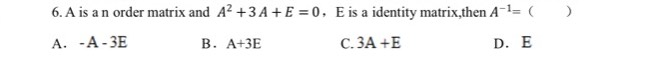### ¿Todavía tienes preguntas de matemáticas?

Pregunte a nuestros tutores expertos
Algebra
PreguntaA is a n order matrix and $$A ^ { 2 } + 3 A + E = 0 , E$$ is a identity matrix, then $$A ^ { - 1 } = ($$ _____

A. $$- A - 3 E$$            B. $$A + 3 E$$                 C. $$3 A + E$$             D. $$E$$

A. $$- A - 3 E$$